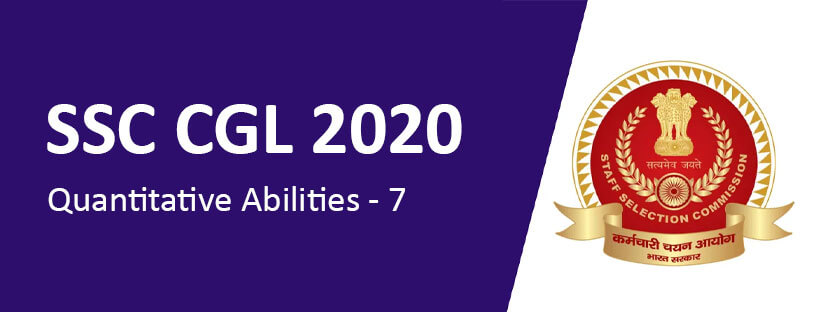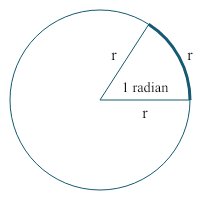SSC CGL 2020: Quantitative Abilities - 7 - Study24x7Default error msg

New to Study24x7 ?
By continuing, you agree to Study24x7 ?

# SSC CGL 2020: Quantitative Abilities - 7

Updated on 26 March 2020SSC Preparation Strategies & S
Updated on 26 March 2020The SSC has online notified SSC CGL new vacancy 2020 the aspirants preparing for competitive exams. The candidates who are targeting for SSC CGL 2020 must go for the give topic below for Quantitative AbilitiesSSC CGL 2020: Degree and Radian Measures

These two measures are used to measure the angles in the field of mathematics.

Degree:

10= 60 minute

Or 1 minute= 10/60If the angle on the center is Ө then

Ө= Length of Arc/ radius of the circle

For example, if the arc length of a circle is 10meter and radius of the circle is 5 meter, then the value of Ө will be

Degree= π/1800

Conversion of 1radian or 1 rad into degree the following process can be used.

For example, n=1

= 1800/3.14 (Take value of π=22/7 or 3.14)

Note: These calculations are used to solve problems on the clock.

SSC CGL 2020: Standard Identities

A standard identity is the one in which the left side of the equation gives the same result as the right-hand side of the equation when variables in the equation are replaced with a constant value.

For example,

Take value of Ө=300

Take the identity sin2Ө + cos2 Ө=1

Put Ө=300 in the equation

sin2300 + cos2 300=1

(1/2)2+ (√3/2)2=1

1/4+3/4=1

4/4=1

1=1

Some of the standard identities of trigonometry are as follows:

1.  sin2Ө+ cos2 Ө=1
2. tan2 Ө+1= sec2 Ө
3. cot2 Ө+1= cosec2
4. sin(-Ө)= -sin Ө
5. cos(-Ө)= cos Ө
6. tan(-Ө)= -tan Ө
7. cosec(-Ө)= - cosec Ө
8. sec(-Ө)=sec Ө
9. cot(-Ө)=-cot Ө
10. tan Ө=sin Ө/cos Ө
11. sec Ө= 1/cos Ө
12. cosec Ө=1/sin Ө

SSC CGL 2020: Complementary Angles

In trigonometry, two angles are said to be complementary if the sum of the two angles is 900.

x and (900-x) are two complementary angles.

For example, we put x=300 than complementary angle of 300 will be (900- 300) = 600

In trigonometry

1. Sin Ө= Cos (900- Ө)

For example, Take Ө=300, take left-hand side of the formula:

sin300=1/2

Right-hand side of the formula:

Cos (900- Ө) = Cos (900- 300)

= Cos 600

=1/2

So the value of both the side of formula is equal.

Likewise,

1. Sin (900- Ө) = Cos Ө
2. Tan (900- Ө) = Cot Ө
3. Cot (900- Ө) = Tan Ө
4. Sec (900- Ө) = Cosec Ө
5. Cosec (900- Ө) = Cosec Ө

All the best to all the SSC CGL Aspirants !

Write a comment...Trending ArticlesBy CBSE CLASS 8By CBSE CLASS 10By CBSE CLASS 7By Edubabble LLP
Related PostsServicesNeed Some Help!Connect